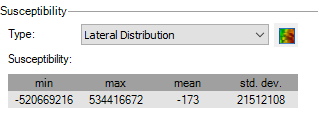# large and negative values in magnetic susceptibility

I converted 4 layer, at various depth, using GMSys-3D. layers at depths of -250, -500, -750, -1000 m depth.
before converting, I used magmap to calculate the apparent magnetic susceptibility for each layer to be used as lateral distribution.

I converted the output from magmap filter to be in MicroCGS for GMSys3D.

results gives me very large numbers of magnetic susceptibility. For example,

Layer (-250) as min= -831.90, Max= 940, mean 18.55, and std.dev= 73.11
Layer (-750) as min= -338340 and max= 3480625, mean= 17, and std.dev= 116327.
Layer (-1000) as min= -520669216, max= 534416672, mean = -173, and std. dev= 21512108 (attached)

is there any explanation for these large and negative numbers.

regards

EssamTagged: